Pilot Courses of Instruction

Aerodynamics

Steve Sconfienza, Ph.D.

Airline Transport Pilot

Flight Instructor: Airplane Single and Multiengine; Instrument Airplane

cell: 518.366.3957

e-mail: docsteve@localnet.com

Deck Angle versus Angle of Attack

It sometimes seems odd to have to explain this, but then again to somebody not instructed in flying this is like magic. First, let's explain "deck angle."

Figure One: Deck Angle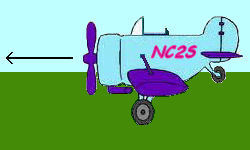# Deck Angle

Assume you are in a normal airplane in a normal place above the earth, let's say some nondescript Cessna at 5,000 feet. You are not in orbit or otherwise high enough to see the curvature of the earth (meaning where the horizon itself is curved, not just looking over the horizon as at a distant mountain or the top of the mast of a sailing vessel coming into port from below the horizon).

Let's say it is a day with more or less good visibility off to the horizon (a few clouds are o.k.): visual meteorological conditions (VMC) [note: VMC (all capital letters) is an entirely different concept from Vmc (capital V and subscript or lower case "mc")]. The wings are level when the port and starboard wings (this is, the left and right wings) are equally spaced with respect to the horizon on either side of the airplane (or other ground reference, but that starts to sound like we're flying into instrument meteorological conditions [IMC], let's stay VFR for now).

All right now, got it? However, not only can the wings be perceived as level (or otherwise) with respect to the horizon, but also the nose as well.

The angle the nose of the aircraft makes with the horizon is the "deck angle," a somewhat nautical sounding term that simply means the angle the nose makes with the deck, that is, the ground (or perhaps the deck of an aircraft carrier?). Nose up is a high deck angle, nose low a low deck angle, and the actual angle with the deck is the angle itself; pitch up 30 degrees above the horizon (which may be read directly from the attitude indicator) is a 30 degree deck angle.

Figure Two: 30 Degree Up Deck Angle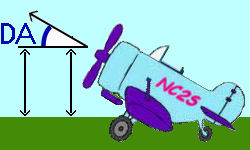Figure Three: 15 Degree Down Deck Angle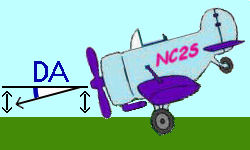In figures one and two above, the straight line parallel to the horizon is just that. The line with the arrow is the direction (up/down) that the nose of the airplane is pointed. Angle DA is the deck angle.

Deck angle is also frequently referred-to as the aircraft's pitch or attitude (attitude as in the round steam gauge on the instrument panel: the "attitude indicator").

Now that that is taken care of, let's take a look at angle of attack.

# Angle of Attack

Angle of attack involves two elements: first, the relative wind, which is the airflow coming towards the aircraft as it flies through the air; and second, the chord-line of the wing, which is the line drawn from the most leading part of the leading edge of the wing to the most trailing part of the trailing edge of the wing. The angle that the relative wind makes with the chord line is the angle of attack. Lift produced by the wing is directly related to the angle of attack. In normal flight conditions (e.g., cruise flight), increasing angle of attack increases lift, while decreasing angle of attack decreases lift.

Figure Four: 15 Degree Angle of Attack, Level Flight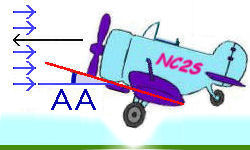In figure three above, the black line with the arrow head is the direction of flight; the red line is the chord line of the wing, extend forward; the blue lines with the arrow heads are the relative wind (opposite the direction of flight), extend to meet the chord line. Angle AA is Angle of Attack.

In figure four below, the above figure is repeated for various flight conditions, — climbs, level flight, and descents — all holding a constant 15° angle of attack.

Figure Five: Constant 15 Degree Angles of Attack
Climbs, Level Flight, Descents

Condition Angle of Attack Deck Angle A. NOTE: Angles are descriptive only and are generally exaggerated well above what would be encountered in actual flight conditions. NOTE: For the aircraft to be in a steady state under each of the above conditions, power would have to be adjusted, from climb power to descent power.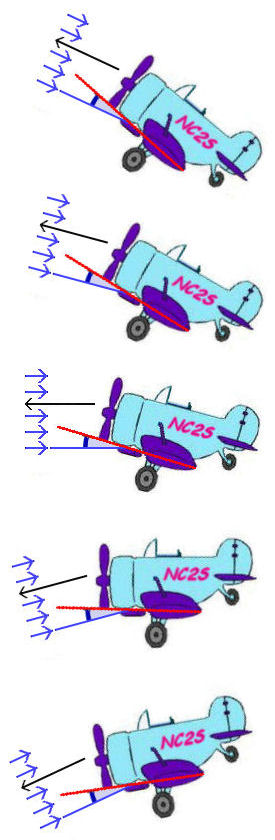Climb 15° 40°(i.e., 40° above the horizon) Climb 15° 30°(i.e., 30° above the horizon) Level Flight 15° 15°(i.e., 15° above the horizon) Descent 15° 0°(i.e., level with the horizon) Descent 15° -15°(i.e., 15° below the horizon)